# 7 ALTERNATIVES TO NESTED IF FUNCTION

IF function is one of the most used functions in Excel.

In my opinion, it is the foundation of all programming and Excel’s formulae mastery.  However, it is also one of the most misused functions, especially Nested IF.

Especially now with Excel 2007 and beyond, you can nest up to 64 IF functions to form complex, slow and hard-to-understand IF THEN ELSE statement.

You don’t need to slow or complicate your worksheet anymore, here are the 7 faster alternatives:

1. VLOOKUP
2. CHOOSE & MATCH
3. REPT
4. INDEX & MATCH
5. SUMPRODUCT
6. BOOLEAN LOGIC
7. SUMIF

## VLOOKUP

Say you have below price list with mixed currency and you want to convert them to USD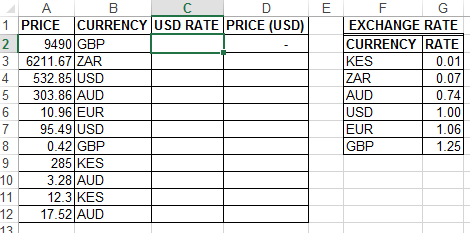The first step would be to look up the exchange rate per currency. Most users would use below nested IF function

`=IF(B2=\$F\$3,\$G\$3,IF(B2=\$F\$4,\$G\$4,IF(B2=\$F\$5,\$G\$5,IF(B2=\$F\$6,\$G\$6,IF(B2=\$F\$7,\$G\$7,IF(B2=\$F\$8,\$G\$8))))))`

This is not only hard to understand but will definitely slow down your worksheet.

Below VLOOKUP Function is the 1st Alternative.`=VLOOKUP(B2,\$F\$2:\$G\$8,2,FALSE)`

How it works

VLOOKUP uses the exact match criteria to retrieve the exchange rates in Column 2 of the exchange rate table (\$F\$2:\$G\$8).

Note: You MUST specify the match criteria for VLOOKUP to exactly retrieve correct rates. IF the criteria is omitted, VLOOKUP, by default, does an approximate search giving wrong results.

## CHOOSE & MATCH FUNCTIONS`=CHOOSE(MATCH(B2,\$F\$3:\$F\$8,0),\$G\$3,\$G\$4,\$G\$5,\$G\$6,\$G\$7,\$G\$8)`

How it works

`MATCH(B2,\$F\$3:\$F\$8,0)=6`

MATCH function searches for the currency in the exchange rate table and returns its relative position.

CHOOSE function returns the exchange rate based on its position in the list. MATCH function above provides this position.

`=CHOOSE(6,\$G\$3,\$G\$4,\$G\$5,\$G\$6,\$G\$7,\$G\$8) = 1.25`

## REPT FUNCTION```=REPT(\$G\$3,B2=\$F\$3)&REPT(\$G\$4,B2=\$F\$4)&REPT(\$G\$5,B2=\$F\$5)
&REPT(\$G\$6,B2=\$F\$6)&REPT(\$G\$7,B2=\$F\$7)&REPT(\$G\$8,B2=\$F\$8)```

How It Works

REPT Function returns a specific text string a specified number of times. If the specified number is zero, REPT returns an empty string.

Excel recognizes internally the value of TRUE as number 1 and value of FALSE as 0. This is a fact that REPT utilizes to determine the number of times to repeat.

Also, note that in our function above ONLY 1 test will evaluate TRUE, others return FALSE.

```=REPT(\$G\$3,FALSE)&REPT(\$G\$4,FALSE)&REPT(\$G\$5,FALSE)
&REPT(\$G\$6,FALSE)&REPT(\$G\$7,FALSE)&REPT(\$G\$8,TRUE)```

Where the test evaluates to FALSE, REPT functions returns an empty string (” “)

```=""&""&""
&""&""&"1.25435"```

## INDEX & MATCH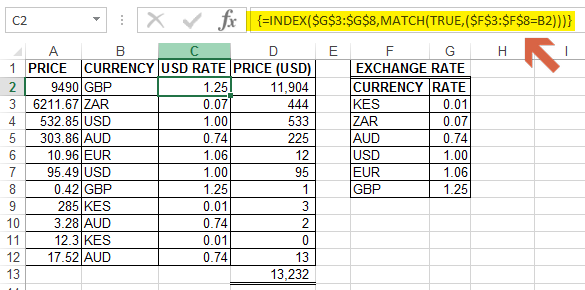`{=INDEX(\$G\$3:\$G\$8,MATCH(TRUE,(\$F\$3:\$F\$8=B2),0))}`

How it works

MATCH function checks if the currency exists in the range (\$F\$3:\$F\$8=B2). If TRUE, it returns the relative position of the currency.

INDEX function returns the exchange rate in the range (\$G\$3:\$G\$8) given its position. MATCH provides this relative position.

NB: This is an array formula, Therefore, Ctrl+Shift+Enter

If want to avoid array formula, use a combination of INDEX, MATCH, INDEX shown below

`=INDEX(\$G\$3:\$G\$8,MATCH(TRUE,INDEX(\$F\$3:\$F\$8=B2,0),0))`

Based on the comment below by Keith, I have realized the above INDEX & MATCH function is an overkill and simple INDEX & MATCH below works perfectly and much faster than above combinations.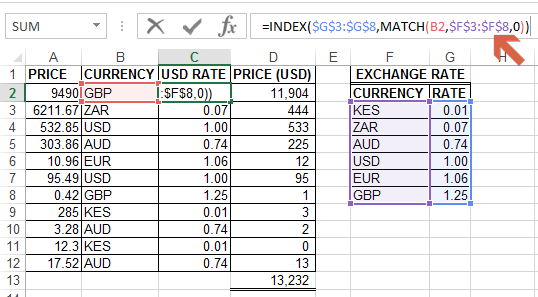`=INDEX(\$G\$3:\$G\$8,MATCH(B2,\$F\$3:\$F\$8,0))`

## SUMPRODUCT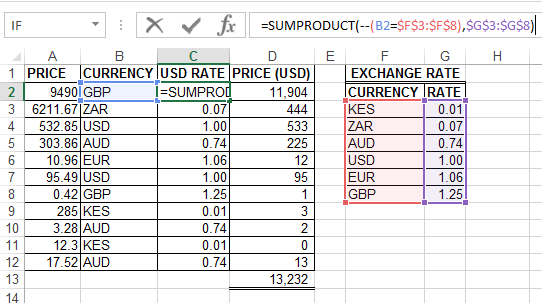`=SUMPRODUCT(--(B2=\$F\$3:\$F\$8),\$G\$3:\$G\$8)`

How it works

In my opinion, this is the shortest and efficient formula.

►–(B2=\$F\$3:\$F\$8) returns an array of 1/0.     {0;0;0;0;0;1}

1 representing the relative position of the currency that meets the criteria in the exchange rate table

►\$G\$3:\$G\$8 returns an array of exchange rates  {0.00982118;0.071507;0.740269;1;1.06172;1.25435}

SUMPRODUCT gets the sum of the product of the two arrays

`=SUMPRODUCT({0;0;0;0;0;1},{0.00982118;0.071507;0.740269;1;1.06172;1.25435})=1.25435`

NB: This method ONLY  works with numeric values.

## BOOLEAN LOGIC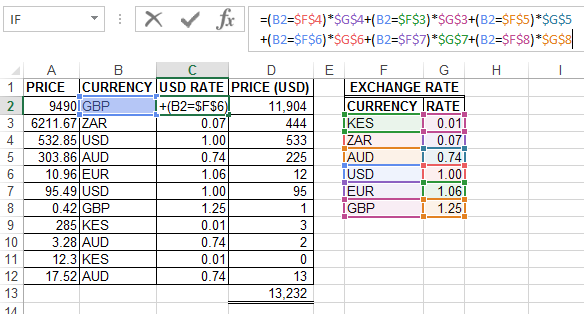```=(B2=\$F\$4)*\$G\$4+(B2=\$F\$3)*\$G\$3+(B2=\$F\$5)*\$G\$5
+(B2=\$F\$6)*\$G\$6+(B2=\$F\$7)*\$G\$7+(B2=\$F\$8)*\$G\$8```

How it works

The method exploits the fact that Excel recognizes internally the value of TRUE as number 1 and value of FALSE as 0.

Firstly, Compare the currency in the price list table with the currency in the exchange rate.  This results to TRUE/FALSE which Excel recognizes as 1/0.

```=(0)*\$G\$4+(0)*\$G\$3+(0)*\$G\$5
+(0)*\$G\$6+(0)*\$G\$7+(1)*\$G\$8```

Since only 1 test will evaluate to TRUE, if you multiply them with corresponding  rates and add them up, the result will be zeros plus the correct rate

```=0+0+0
+0+0+1.25435```

NB: This method also ONLY  works with numeric values.

## SUMIFHow it works

This is another simple alternative but works ONLY with numeric values.

SUMIF function returns a sum of all numbers in a range of cells that meet a certain criterion.

syntax =SUMIF(Range, Criterion, [sum_range])

Range–Currencies to be evaluated using our criterion

Criterion–the currency on our price list that determines the exchange rate to be returned

Sum_range–the exchange rates

Since only one cell in our range will meet our criterion, SUMIF returns the coinciding rate.

### CONCLUSION

There you have it–7 ways to eliminate the slow calculating and complex nested IF.

### RELATED ARTICLES

7 OVERLOOKED USES OF EXCEL MOD FUNCTION

EXCEL’S WEEKDAY FUNCTION IN DEPTH

### RESOURCES

This Article was inspired the Excel Hero epic article  I Heart IF.

### RECOMMENDED BOOKS

EXCEL OUT OF BOX CREATIVITY BOOKS

## 15 thoughts on “7 ALTERNATIVES TO NESTED IF FUNCTION”

1.Suzana Stojadinovic Pavlovic says:

Дивно објашњено. Да ли исти проблем може да се реши хоризонталном функцијом HLOOKUP.

1.Crispo Mwangi says:

Suzana,
Ја бих радије да користите индек & меч за хоризонтални лоокуп

2.Gijs Michels says:

I have also an idea with vba you can make you own function aafter that you can write it simple in a macro

example
dim x as string
for i =2 to 25
x=range(“A” & I)
Range (“b” & i).value =myfunction(x)
next i
If you want to know how you make your own function i will write you an example with explains how to organize it.

1.Crispo Mwangi says:

Thanks Gijs for the comment.

3.Keith says:

My very first thought is an Index & Match that is at its simplest. You have two others that are more complicated. This formula will work as well.
=INDEX(\$G\$3:\$G\$8, MATCH(B2,\$F\$3:\$F\$8,0))

1.Crispo Mwangi says:

Keith,
Very True! Your suggestion works well.
I fell into the trap of Overthinking the solution!

Thanks for noting this!

4.Ajay says:

The INDEX MATCH function suits & easiest plus accurate method

5.William says:

Cool techniques. I have not thought of SUMPRODUCT in that light as an alternative to VLOOKUP.

1.Crispo Mwangi says:

Glad you have learned something new!

6.Pierre-Louis says:

As usual, very interesting technics/formulas to use!
And as per William’s comment, I love the SUMPRODUCT as an alternative to VLOOKUP.

1.Crispo Mwangi says:

Thanks, Pierre.

My favorites are SUMPRODUCT and SUMIF.

7.Imrod Kartiko says:

Thanks for sharing..it helps me thinking using others function solution rathet than using complex and long nested if….

1.Crispo Mwangi says:

Thanks, Imrod.

8.David says:
1.Crispo Mwangi says: